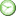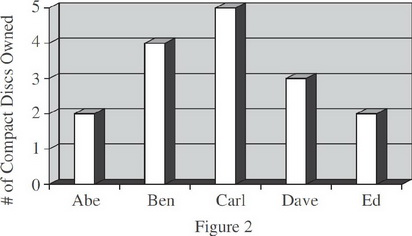# ISEE Math Practice Test: Charts and Graphs

### Test Information8 questions0 minutes

Take more free ISEE Mathematics Achievement Practice Tests available from crackssat.com.

1. Question below refer to the following summary of energy costs by district.

 District 2013 2014 A 400 600 B 500 700 C 200 350 D 100 150 E 600 800 (All numbers are in thousands of dollars.)

In 2014, which district spent twice as much on energy as District A spent in 2013 ?

• A. A
• B. B
• C. C
• D. E

2. Question below refer to the following summary of energy costs by district.

 District 2013 2014 A 400 600 B 500 700 C 200 350 D 100 150 E 600 800 (All numbers are in thousands of dollars.)

Which district spent the most on electricity in 2013 and 2014 combined?

• A. A
• B. B
• C. D
• D. E

3. Question below refer to the following summary of energy costs by district.

 District 2013 2014 A 400 600 B 500 700 C 200 350 D 100 150 E 600 800 (All numbers are in thousands of dollars.)

The total increase in energy expenditure in these districts, from 2013 to 2014, is how many dollars?

• A. \$800
• B. \$1,800
• C. \$2,600
• D. \$800,000

4. Question below refers to Figure 2, which shows the number of compact discs owned by five students.Carl owns as many CDs as which two other students combined?

• A. Abe and Ben
• B. Ben and Dave
• C. Abe and Ed
• D. Abe and Dave

5. Question below refers to Figure 2, which shows the number of compact discs owned by five students.Which one student owns one-fourth of the CDs accounted for in Figure 2 ?

• A. Abe
• B. Ben
• C. Carl
• D. Dave

6. Question below refers to Matt's weekly time card, shown below.If Matt's hourly salary is \$6, what were his earnings for the week?

• A. \$14
• B. \$21
• C. \$54
• D. \$84

7. Question below refers to Matt's weekly time card, shown below.What is the average number of hours Matt worked on the days he worked during this particular week?

• A. 3
• B. 3.5
• C. 4
• D. 7

8. Question below refers to Matt's weekly time card, shown below.The hours that Matt worked on Monday accounted for what percent of the total number of hours he worked during this week?

• A. 3.5
• B. 20
• C. 25
• D. 35